Get Started »

# Algebra

You can factor or expand algebraic expressions:

(Use CTRL+6 for typeset exponents.)
 In:=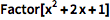⨯ `Factor[x^2 + 2 x + 1]`
 Out=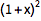The Wolfram Language uses == (two equal signs) to test equality:

 In:=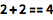⨯ `2 + 2 == 4`
 Out=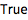Combine algebraic expressions with == to represent an equation:

 In:=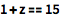⨯ `1 + z == 15`
 Out=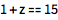Commands like Solve find exact solutions to equations:

 In:=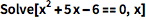⨯ `Solve[x^2 + 5 x - 6 == 0, x]`
 Out=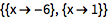For approximate results, use NSolve:

 In:=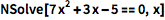⨯ `NSolve[7 x^2 + 3 x - 5 == 0, x]`
 Out=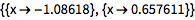Pass in a system of equations as a list:

 In:=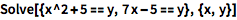⨯ `Solve[{x^2 + 5 == y, 7 x - 5 == y}, {x, y}]`
 Out=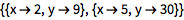Find the roots of an equation:

(|| is the symbol for Or.)
 In:=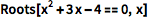⨯ `Roots[x^2 + 3 x - 4 == 0, x]`
 Out=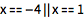If a polynomial is not easily factorable, approximate results may be more useful:

 In:=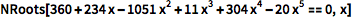⨯ `NRoots[360 + 234 x - 1051 x^2 + 11 x^3 + 304 x^4 - 20 x^5 == 0, x]`
 Out=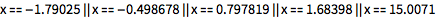The Reduce command reduces a set of inequalities into a simple form:

(Type <= for the `≤` symbol.)
 In:=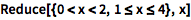⨯ `Reduce[{0 < x < 2, 1 <= x <= 4}, x]`
 Out=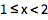The reduced form may include multiple intervals:

 In:=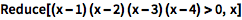⨯ `Reduce[(x - 1) (x - 2) (x - 3) (x - 4) > 0, x]`
 Out=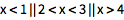NumberLinePlot is a handy way to visualize these results:

 In:=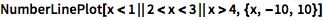⨯ `NumberLinePlot[x < 1 || 2 < x < 3 || x > 4, {x, -10, 10}]`
 Out=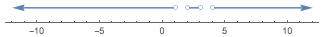Many equations and formulas are available through natural-language input:

 In:=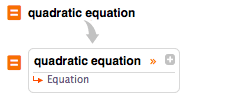X `quadratic equation`
 Out=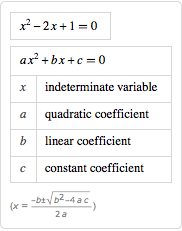QUICK REFERENCE: Polynomial Equations `»`

QUICK REFERENCE: Equation Solving `»`